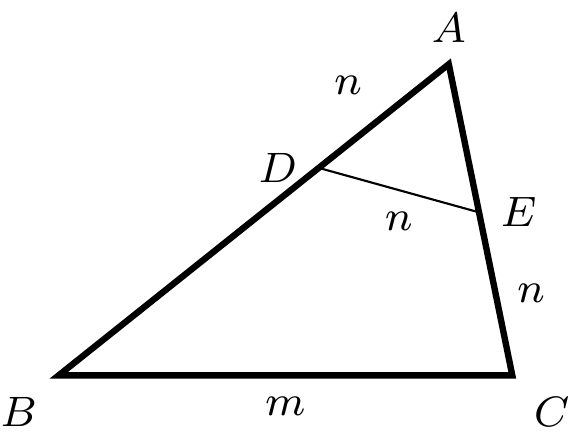#### Switzerland

###### back to index

In $\triangle{ABC}$, $AB = 33, AC=21,$ and $BC=m$ where $m$ is an integer. There exist points $D$ and $E$ on $AB$ and $AC$, respectively, such that $AD=DE=EC=n$ where $n$ is also an integer. Find all the possible values of $m$.